{{ message }}

# holoviz / holoviews

Merged
merged 28 commits into from Dec 14, 2017
Merged

merged 28 commits into from Dec 14, 2017

## Conversation

###philippjfr commented Nov 22, 2017 • edited by jbednar

 Triangle meshes are a common form of data when working with complex polygons and are frequently employed in environmental modeling. The most common way to compute such a mesh is using Delaunay triangulation and the data is usually represented as two data structures: The simplices representing each triangle, usually these are node indices. The nodes or points representing each corner of a triangle. This representation is in fact simply a specific type of graph and closely follow the data structures we already use for graphs, where the simplices represent the abstract connectivity of the mesh and the nodes represent the positions. It was therefore trivial to write a `TriMesh` element which simply reuses the same data structures, organization, and plotting code as existing graph elements. Here is a simple example: ```import param import numpy as np import holoviews as hv from scipy.spatial import Delaunay from holoviews.element.graphs import Graph, EdgePaths hv.extension('bokeh') n_verts = 1000 pts = np.random.randint(1, n_verts, (n_verts, 2)) tris = Delaunay(pts) hv.TriMesh((tris.simplices, tris.points))```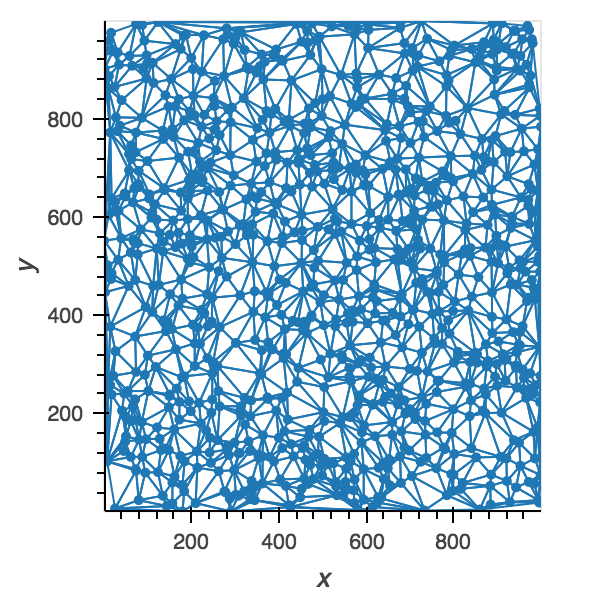This approach is reasonably fast for small meshes (~1 second/5000 triangles) and once you start plotting more than ~10k triangles you will want to use datashader anyway. It is also flexible enough to associate additional values both with the simplices and with the nodes. Once holoviz/datashader#525 is merged I will get on with allowing datashader operation such as aggregate to operate on the TriMesh element. Reference gallery entries Unit tests

###philippjfr commented Nov 23, 2017 • edited

 If you enable WebGL rendering in bokeh you can actually scale up to some pretty large meshes, here is an example of selecting on 40485 triangles: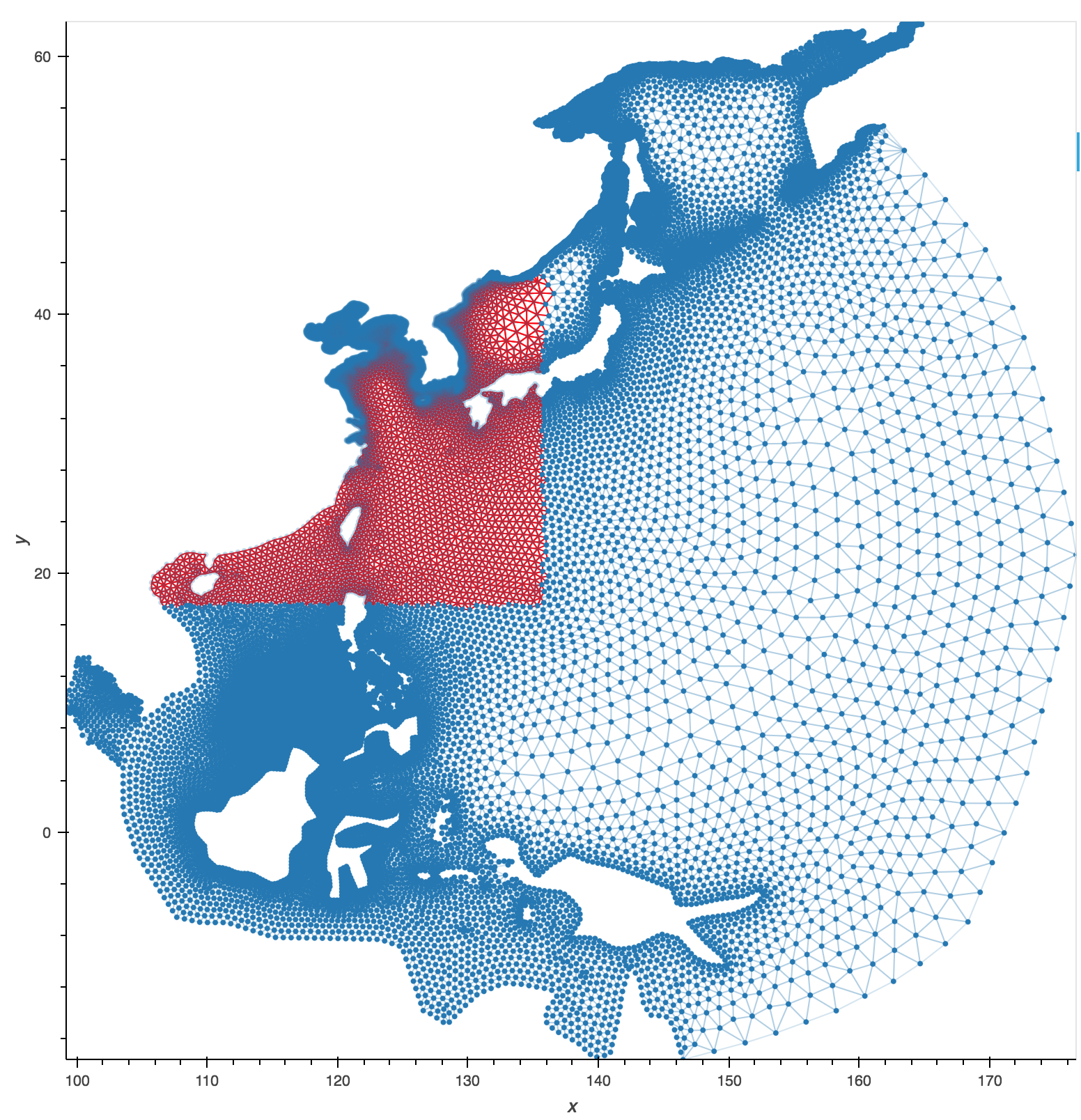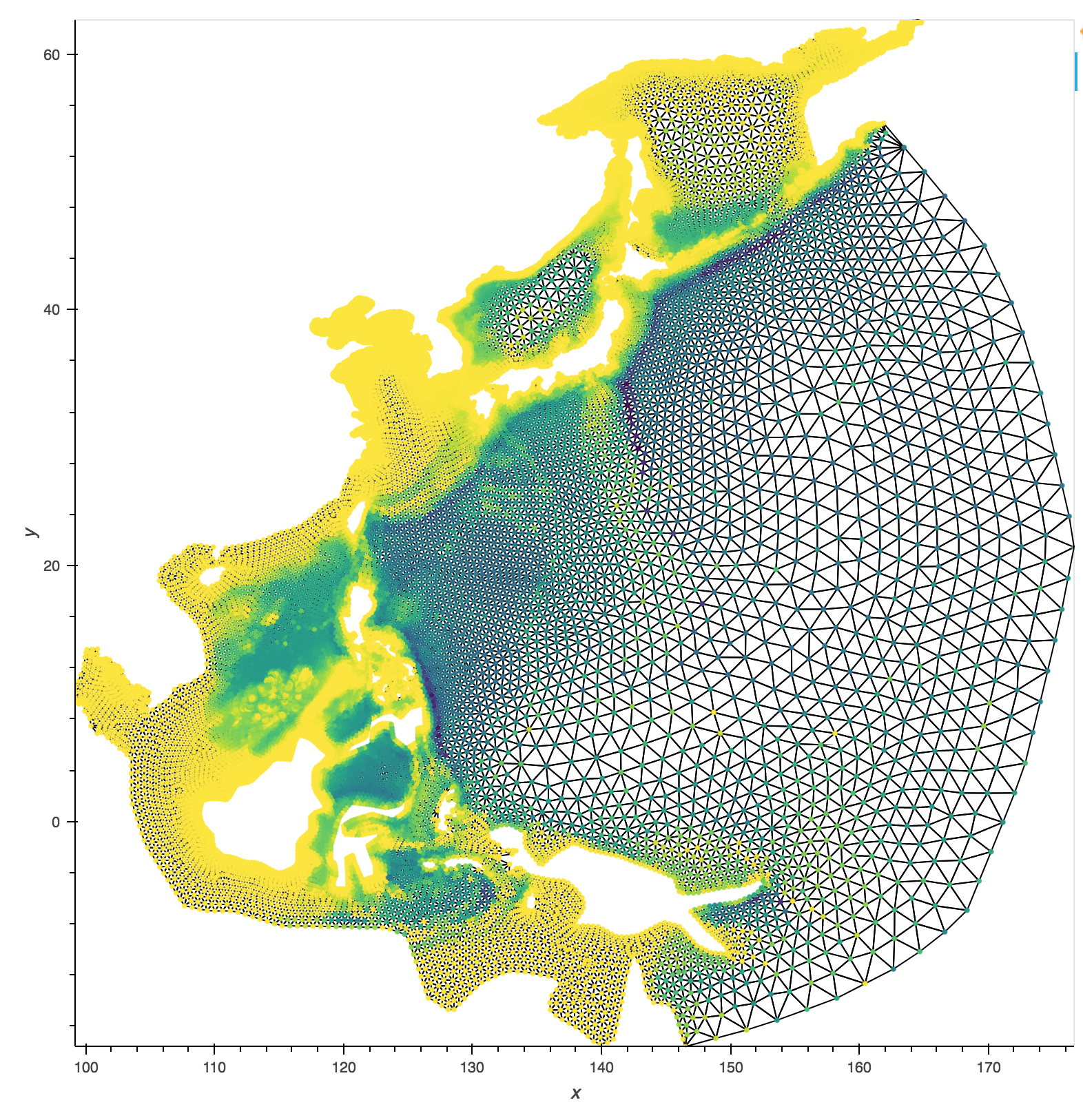###philippjfr commented Nov 24, 2017

 I've now allowed filling the triangles by a value, so here's the example above this time with filled triangles: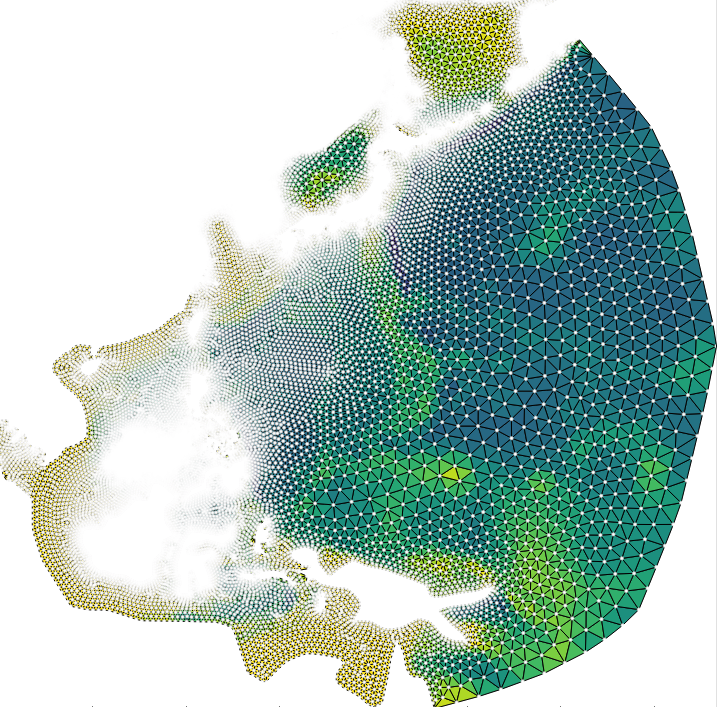I'd now consider this PR ready for review, I've added unit tests and reference notebooks. The datashading portion will follow in another PR once it's been merged into datashader.

###philippjfr commented Nov 24, 2017

 Yay, we even gained coverage.

###basnijholt commented Dec 2, 2017

 I am trying this out since I work a lot with triangulations, currently, I create plots like: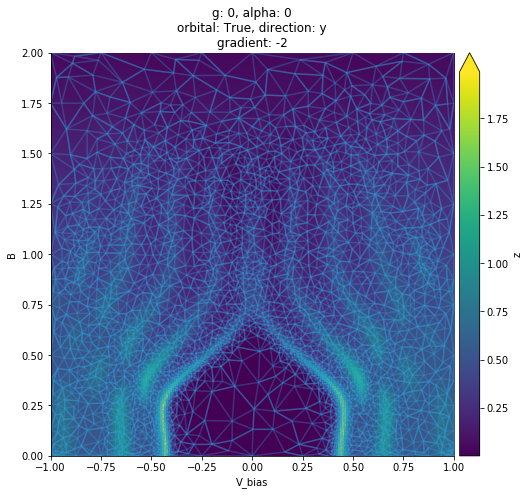(an Overlay of Polygon and Image) Using this branch I am trying to get something similar, but it's not getting better: ```%%output size=200 %%opts TriMesh (node_size=0 edge_line_width=0.1 edge_nonselection_alpha=0.01) plot * hv.TriMesh((tris.simplices, points))```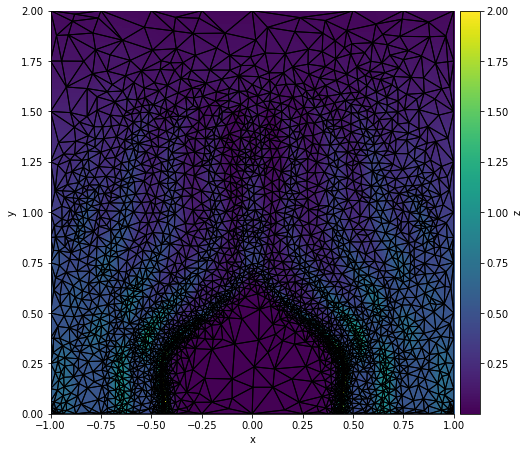I am unable to set the `alpha` and `linewidth`, am I not understanding it, or is this a bug?

###basnijholt commented Dec 2, 2017 • edited

 Another question, the `hv.Image` I used in the `Overlay`s above are created from an interpolation that uses the `scipy.spatial.Delaunay` object, but looking at your example plot it seems to be possible to fill the triangles directly with the values at the vertices, but I can't see how exactly. Could you please give a simple example?

###philippjfr commented Dec 3, 2017

 Looks like you're using the matplotlib backend but specifying bokeh options, try changing that to `edge_linewidth` and `edge_alpha`. but looking at your example plot it seems to be possible to fill the triangles directly with the values at the vertices, but I can't see how exactly. Have a look at the example notebook, the last example demonstrates it. Going to be pushing a small fix for that shortly.

###philippjfr commented Dec 8, 2017

 @jlstevens @jbednar Requesting review.
approved these changes

###jbednar left a comment

 Looks great!
 Element or overlay of Elements into an hv.Image or an overlay of hv.Images by rasterizing it, which provides a fixed-sized Element or overlay of Elements into an hImage or an overlay of .Images by rasterizing it, which provides a fixed-sized

#### jbednar Dec 8, 2017 Member

What are hImage and .Image ?

 return Image(agg, **params) class rasterize(trimesh_rasterize):

#### jbednar Dec 8, 2017 Member

How can rasterize be derived from trimesh_rasterize? That seems like a broken type hierarchy, as it does not satisfy "is a" ("rasterize" is not a kind of "trimesh_rasterize").

#### philippjfr Dec 8, 2017 • edited Author Member

True, it was easiest to inherit all the methods and parameters but a cleaner class hierarchy is worth the extra handling.

 (not isinstance(x, Image) or x in imgs)) element = element.map(dsrasterize, predicate) return element

#### jbednar Dec 8, 2017 Member

Does this not yet handle regridding?

#### philippjfr Dec 8, 2017 • edited Author Member

I was going to handle that in another PR once datashader has made the API more consistent.

#### jbednar Dec 8, 2017 Member

Ok. And that's on my plate, but after this conference prep...

###basnijholt commented Dec 8, 2017 • edited

 Great work! 👍 How can I use the interpolation here? How do I go from: ```%%opts TriMesh [filled=True edge_color_index='z'] (cmap='viridis' node_alpha=0 edge_line_alpha=0.4) def plot_TriMesh(learner): ip = learner.ip() simplices = ip.tri.simplices nodes = ip.tri.points z = ip.values[simplices].mean(axis=1) return hv.TriMesh((np.column_stack([simplices, z]), nodes), vdims='z') plot_TriMesh(learner)```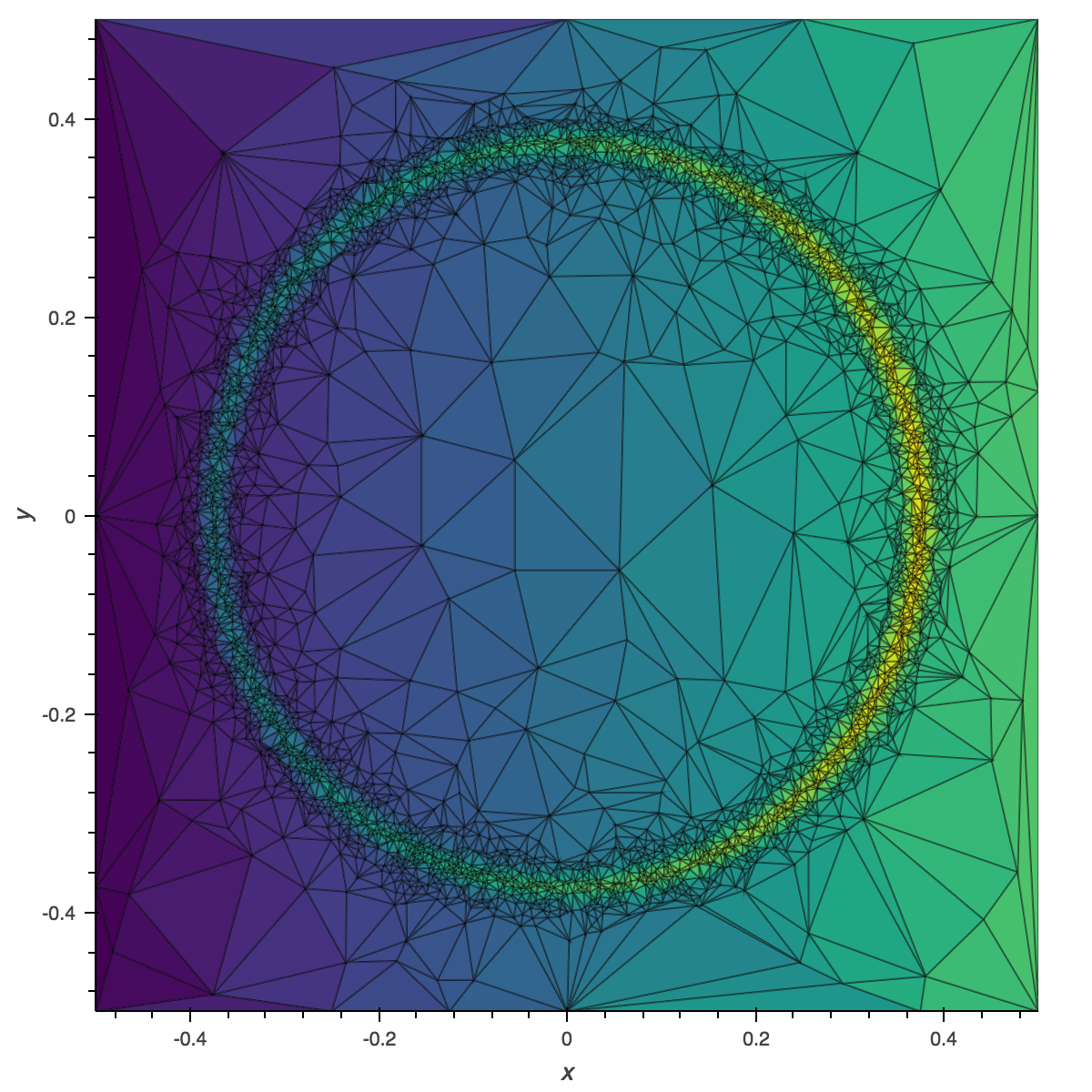to ``````%%opts Image (cmap='viridis') %%opts Contours (color='k') learner.plot(triangles_alpha=0.4) ``````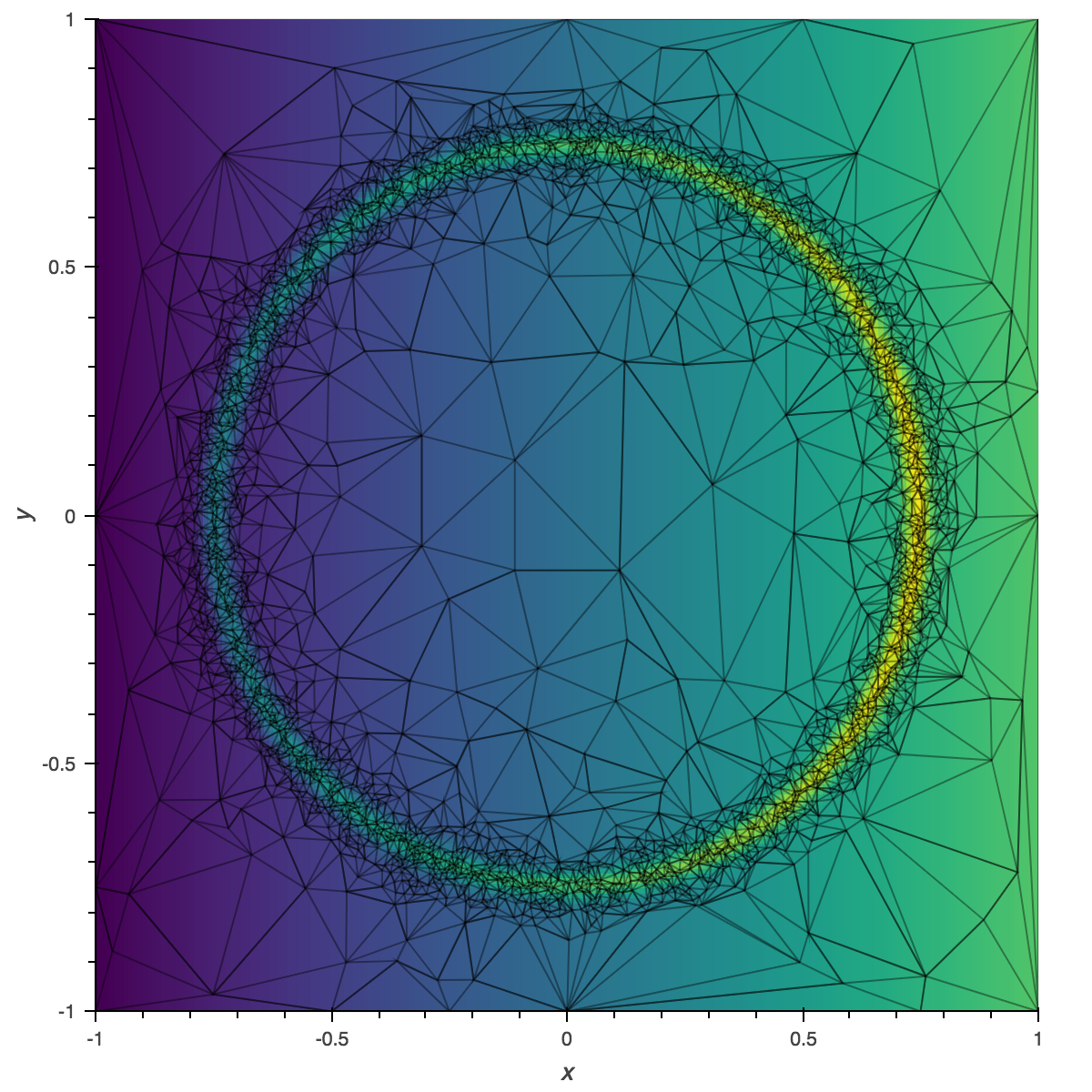I would like to know because: ```%timeit plot_TriMesh(learner) 2.71 ms ± 309 µs per loop (mean ± std. dev. of 7 runs, 100 loops each) %timeit learner.plot(triangles_alpha=0.4) 216 ms ± 13.9 ms per loop (mean ± std. dev. of 7 runs, 1 loop each)``` EDIT the timing is not such an issue anymore, implemented an overlay of an `Image` and `TriMesh` ``````# new %timeit learner.plot(triangles_alpha=0.4) 11.7 ms ± 803 µs per loop (mean ± std. dev. of 7 runs, 100 loops each) # old %timeit learner.plot(triangles_alpha=0.4) 317 ms ± 17.3 ms per loop (mean ± std. dev. of 7 runs, 1 loop each) `````` however `learner.plot()` now fails when there is no data yet.

###basnijholt commented Dec 8, 2017 • edited

 For the same reason `hv.Image([])` is useful, the same would go for `hv.TriMesh`. Would it be easy to make this work with `hv.TriMesh(([], []))`or something? My data is calculated and plotted live, and in be beginning there might not be data to plot yet. Then I could write beautiful things like: ```if self.data: x = y = np.linspace(-0.5, 0.5, n) ip = self.ip() z = ip(x[:, None], y[None, :]) image = hv.Image(z, bounds=lbrt) tris = hv.TriMesh((ip.tri.simplices, self.unscale(ip.tri.points))) tris = tris.opts(style=dict(edge_line_alpha=triangles_alpha)) plot = image * (tris if triangles_alpha else hv.TriMesh(([], []))) else: plot = hv.Image([]) * hv.TriMesh(([], []))```

###philippjfr commented Dec 9, 2017

 @basnijholt Both `hv.TriMesh(([], []))` and `hv.TriMesh([])` now work.

###basnijholt commented Dec 9, 2017

 How can I change the colors of the edges? (I think) I've tried all style parameters with `*_color*` but I am not able to get it to work.

###philippjfr commented Dec 13, 2017

 @basnijholt Are your values on the vertices or simplexes? Currently it only allows coloring by values on the simplexes.

###philippjfr commented Dec 13, 2017

 @jlstevens Requesting review again.

###basnijholt commented Dec 13, 2017

 Are your values on the vertices or simplexes The values are at the vertices.
reviewed
 """ Rasterize is a high-level operation which will rasterize any Element or combination of Elements supplied as an (Nd)Overlay by aggregating with the supplied aggregation it with the declared

#### philippjfr Dec 13, 2017 Author Member

Need to fix this docstring.

reviewed
 supplied, which will ensure the selection is only applied if the specs match the selected object. """ self.edgepaths

#### philippjfr Dec 13, 2017 Author Member

Will add a comment about this. By default `edgepaths` are not computed but .select expects it to be.

#### jlstevens Dec 13, 2017 Contributor

Yes, a comment next to this line explaining it would be a good idea.

added 23 commits Nov 24, 2017
``` Added support for filled TriMesh simplices ```
``` 63067ce ```
``` Defined default TriMesh styling ```
``` c6e9146 ```
``` Small fix for TriMesh path computation ```
``` 3f61cca ```
``` Added TriMesh reference entries ```
``` bcbb7bf ```
``` Added tests and small fixes for TriMesh element ```
``` bdbb3cc ```
``` Various TriMesh improvements ```
``` 58b98b0 ```
``` Added trimesh rasterization and generalized API ```
``` 2dcbc26 ```
``` Split matplotlib paths before displaying them ```
``` d16a637 ```
``` Optimized static bokeh Graph plots ```
``` d5a6ea6 ```
``` Fixed various Graph edgepaths bugs ```
``` 3f2a28d ```
``` Restored default datashading aggregator ```
``` d8cfd66 ```
``` Updated unit test ```
``` 1c9585d ```
``` Improved datashader dimension lookup ```
``` 9f9b569 ```
``` Added interpolation support for trimesh rasterization ```
``` b7f04ae ```
``` Improved datashader operations ```
``` 635a8ce ```
``` Improvements for bokeh GraphPlot ```
``` cf0d3c4 ```
``` Cleaned up datashader class hierarchy ```
``` 2243bf6 ```
``` Handled empty TriMesh ```
``` 586a506 ```
``` Addressed various TriMesh review comments ```
``` 3480316 ```
``` Fixed Dataset.add_dimension dtype bug ```
``` ad2fcca ```
``` Fixed datashade operation docstrings ```
``` 74f961a ```
``` Added vertex averaging to TriMesh plots ```
``` 65a44bd ```
``` Revised TriMesh reference notebooks ```
``` ddf38cd ```